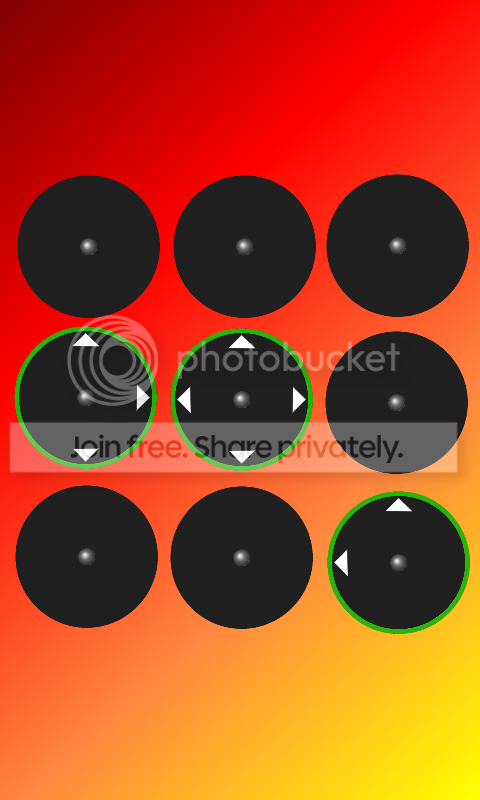-=+=- -=+=- -=+=- -=+=- -=+=- -=+=- -=+=- -=+=- -=+=- -=+=- -=+=- -=+=- -=+=- -=+=- -=+=- -=+=- -=+=- -=+=- -=+=- -=+=- -=+=- -=+=- -=+=- -=+=- -=+=- -=+=- -=+=- -=+=- -=+=- -=+=- (c) WidthPadding Industries 1987 0|533|0 -=+=- -=+=- -=+=- -=+=- -=+=- -=+=- -=+=- -=+=- -=+=- -=+=- -=+=- -=+=- -=+=- -=+=- -=+=- -=+=- -=+=- -=+=- -=+=- -=+=- -=+=- -=+=- -=+=- -=+=- -=+=- -=+=- -=+=- -=+=- -=+=- -=+=-
SoCoder -> Showcase Home -> Puzzle

9572ADCreated : 01 April 2012
Edited : 02 April 2012
System : Amstrad CPC
Language : Forth

### Nuthin' But An droid Thing

a phoney puzzle

ScreenshotsA "Lights Out" variant that looks similar to an Android phone unlock screen.

### Comments

Monday, 02 April 2012, 04:39
JayenkaiSounds like a plan.

... although having ads will probably result in a much better income.
Take it from someone who's had minimal at best sales..